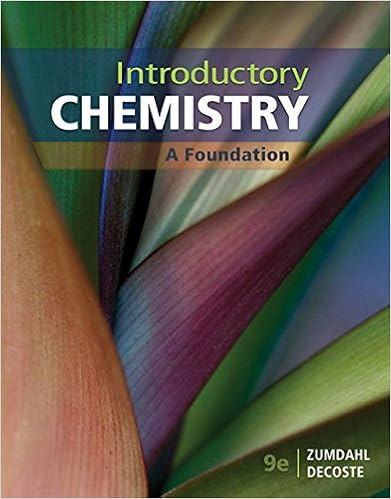# Points The function E x is defined by E x ...

• Notes
• 7

This preview shows page 1 - 7 out of 7 pages.

##### We have textbook solutions for you!
The document you are viewing contains questions related to this textbook.The document you are viewing contains questions related to this textbook.
Chapter 17 / Exercise 23
Introductory Chemistry: A Foundation
ZumdahlExpert Verified
Name: Section Number: TA Name: Section Time: Math 10B. Final Examination March 16, 2004 Read each question carefully, and answer each question completely. Show all of your work. No credit will be given for unsupported answers. Write your solutions clearly and legibly. No credit will be given for illegible solutions. 1. (6 points) The function E ( x ) is defined by E ( x ) = 1 2 + 1 2 π x 0 e - t 2 2 dt . (a) Compute E (0). (b) Compute E (0). (c) Compute E (1). # Score 1 2 3 4 5 6 7 8 9 Σ
##### We have textbook solutions for you!
The document you are viewing contains questions related to this textbook.The document you are viewing contains questions related to this textbook.
Chapter 17 / Exercise 23
Introductory Chemistry: A Foundation
ZumdahlExpert Verified
2. (8 points) Compute the following definite integrals. (a) 1 0 xe x 2 dx (b) 1 0 x 3 e x 2 dx
3. (4 points) Use the Trapezoidal Rule with four subintervals ( n = 4) to estimate 1 + x 3 dx . Do not simplify the resulting expression. 4 0 1 4. (4 points) Determine whether x - 5 2 dx converges, and if it does, find its value. 1
5. (4 points) After conducting extensive research, the American Automobile Association has discovered that the length of time between tuneups for minivans is a continuous random variable T , measured in years, and that the probability density function for T is f ( T ) = 0 if T < 0 1 9 Te - T 3 if T 0 What is the probability that a minivan will be driven between one and two years
•••